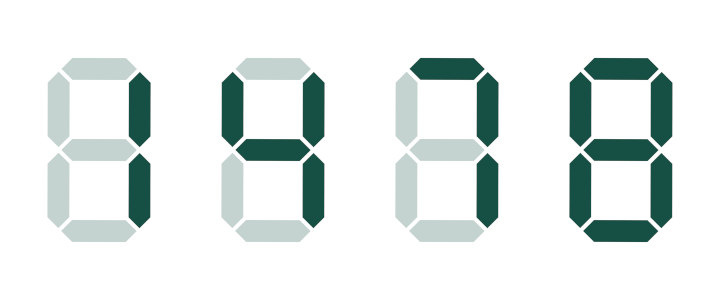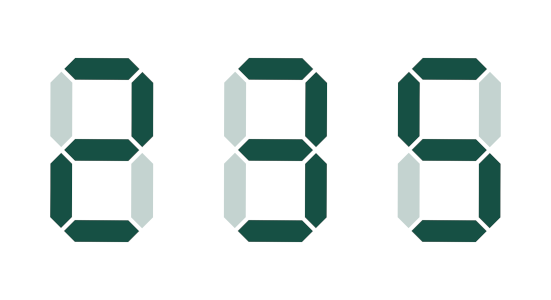# Advent of Code 2021 - Day 8

By Eric Burden | December 8, 2021

It’s that time of year again! Just like last year, I’ll be posting my solutions to the Advent of Code puzzles. This year, I’ll be solving the puzzles in Julia. I’ll post my solutions and code to GitHub as well. I had a blast with AoC last year, first in R, then as a set of exercises for learning Rust, so I’m really excited to see what this year’s puzzles will bring. If you haven’t given AoC a try, I encourage you to do so along with me!

# Day 8 - Seven Segment Search

Find the problem description HERE.

## The Input - Guess Who’s Back

While today’s input format isn’t super complicated, we’ll be doing a good amount of parsing and manipulating that input, so it makes sense to ingest it as an efficient data type. I’ve found that working with Tuples generally results in faster code than using structs, where it makes sense. Since we know how many values we’ll have in each input record, we can use NTuples for each part.

const SignalPatterns = NTuple{10,String}
const RawOutputs = NTuple{4,String}
const RawInput = @NamedTuple {patterns::SignalPatterns, outputs::RawOutputs}

function parseline(s::AbstractString)::RawInput
(patternstr, outputstr) = split(s, " | ")
sortstr = join ∘ sort ∘ collect
patterns = Tuple([sortstr(s) for s in split(patternstr)])
outputs = Tuple([sortstr(s) for s in split(outputstr)])
return (patterns = patterns, outputs = outputs)
end

function ingest(path)
return open(path) do f
end
end


## Part One - Easy Street

Today’s puzzle has us decoding scrambled seven-segment displays. Quite frankly, I’m appalled at the missed opportunity to have this be Day 7’s puzzle, but you don’t always get what you want, as a wise man once said. It looks like our submarine has, like, a bajillion of these seven segment displays, each displaying four digits of pure chaotic garbage at the moment, and our job is to unscramble them. Well, that’s probably our job in part two. Our job in part one is just to figure out how many times the numbers 1, 4, 7, and 8 appear in our display outputs. This is somewhat easy, because each of these numbers contains a unique count of the seven segments, like so:All other numbers, it turns out, are represented by either five or six segments. So, for this part, we just look over all the output values (the ones to the right of the | in the puzzle input), and count the number of strings of length 2, 4, 3, or 8.

# Given a RawOutput (a Tuple of letter combinations), identify which
# ones represent a number containing a unique number of segments, and
# just count how many you found.
function counteasydigits(outputs::RawOutputs)::Int
easyfilter(output) = filter(x -> length(x) in [2, 3, 4, 7], output)
(outputs
|> easyfilter
|> length)
end

# Search for the obvious number displays in our outputs and count the
# number found.
function part1(input)
tooutputs(RI) = map(x -> x.outputs, RI)
easycount(input) = map(counteasydigits, input)
(input
|> tooutputs
|> easycount
|> sum)
end


So, that wasn’t too difficult. In fact, it was almost suspiciously easy…

## Part Two - Set Me Up

Now we’re paying for the relatively simple part one, and to be honest, we definitely should have seen this one coming. Now our main job is to decode the rest of the signals to identify which combination of letters corresponds to each display of a particular number. To do this, we’ll compare the letters corresponding to the segments in the display of known numbers (1, 4, 7, 8) to the letters corresponding to the segments in the display of unknown numbers (0, 2, 3, 5, 6, 9) to deduce the unknown numbers. We know how many segments are in each of the unknown number displays (5 segments -> 2, 3, 5; 6 segments -> 0, 6, 9), so armed with these known lengths and our four known collections of segments, we can deduce the remaining numbers. In our solution below, we start with the 5-length signals, then move on to the 6-length signals. For your reference, here are the segment arrangements that represent the rest of the numbers:One example of our approach is identifying the segments that represent the number 3. Of the 5-segment combinations, only 3 contains all the segments from the display of 1. Using rules like this for all 6 of the unknown numbers allows us to identify them and get the problem solution, which is to use our known signal -> number mapping to get the total value of each of the outputs and sum them together to solve the puzzle.

function decode(rawinput::RawInput)::Int
(patterns, outputs) = rawinput

# Sort the patterns such that the unique patterns are placed in the front
# by length, and the non-unique patterns are sorted to the back.
sortpatternsby(x) = length(x) in [5, 6] ? length(x) + 5 : length(x)
sortedpatterns = sort(collect(patterns), by = sortpatternsby)

# These are the easy matches, sorted to the front of
# sortedpatterns by our sorting function
patternmatches = [
(sortedpatterns, 1),
(sortedpatterns, 7),
(sortedpatterns, 4),
(sortedpatterns, 8)
]

# Match the 5- and 6-length patterns
(one, seven, four) = sortedpatterns[1:3]
fivecharmatches = getfivecharmatches(sortedpatterns[5:7], four, seven)
sixcharmatches  = getsixcharmatches(sortedpatterns[8:10], four, seven)
append!(patternmatches, fivecharmatches, sixcharmatches)

# Now with all the patterns deciphered, let's get the
# values in our outputs
patterndict = Dict(patternmatches)
numbers = reverse([patterndict[output] for output in outputs])
return sum(numbers[i] * 10^(i - 1) for i = 1:length(numbers))
end

function getfivecharmatches(patterns, four, seven)::Vector{Tuple{String,Int}}
matches = []

# Use a set of letters representing the segments left if you "subtract"
# the segments in seven from the segments in four to help find the
# length 5 displays
fournotseven = setdiff(four, seven)

# Rules to identify two, three, and five
istwo(x)   = seven ⊈ x && fournotseven ⊈ x
isthree(x) = seven ⊆ x
isfive(x)  = fournotseven ⊆ x

# Check each length 5 set of segments and identify two, three, and five
for pattern in patterns
match = istwo(pattern)   ? (pattern, 2) :
isthree(pattern) ? (pattern, 3) :
isfive(pattern)  ? (pattern, 5) : missing
ismissing(match) && error("Could not match pattern $pattern") push!(matches, match) end return matches end function getsixcharmatches(patterns, four, seven)::Vector{Tuple{String,Int}} matches = [] # Rules to identify zero, six, and nine based on known displays iszero(x) = four ⊈ x && seven ⊆ x issix(x) = four ⊈ x && seven ⊈ x isnine(x) = four ⊆ x && seven ⊆ x # Check each length 6 set of segments and identify zero, six, and nine for pattern in patterns match = iszero(pattern) ? (pattern, 0) : issix(pattern) ? (pattern, 6) : isnine(pattern) ? (pattern, 9) : missing ismissing(match) && error("Could not match pattern$pattern")
push!(matches, match)
end
return matches
end

function part2(input)
decodeeach(x) = map(decode, x)
(input
|> decodeeach
|> sum)
end


That’s kind of a lot of code, but each 5- and 6-segment display number has to have it’s own rule for identification. This code, at least, manages to make all the identifications in a single pass over the sorted signals. My first crack at this took three separate passes.

## Wrap Up

Hoo boy, today was something. Once I realized I could deduce the unknown numeric displays from the known displays, it really became a matter of trying to figure out which order to decode each unknown signal in, and a mental exercise in whether I could use just the “easy” signals to decode the rest. All in all, this was a more challenging puzzle that gave me a chance to use (and learn) some of the set notation operators in Julia.

If you found a different solution (or spotted a mistake in one of mine), please drop me a line!